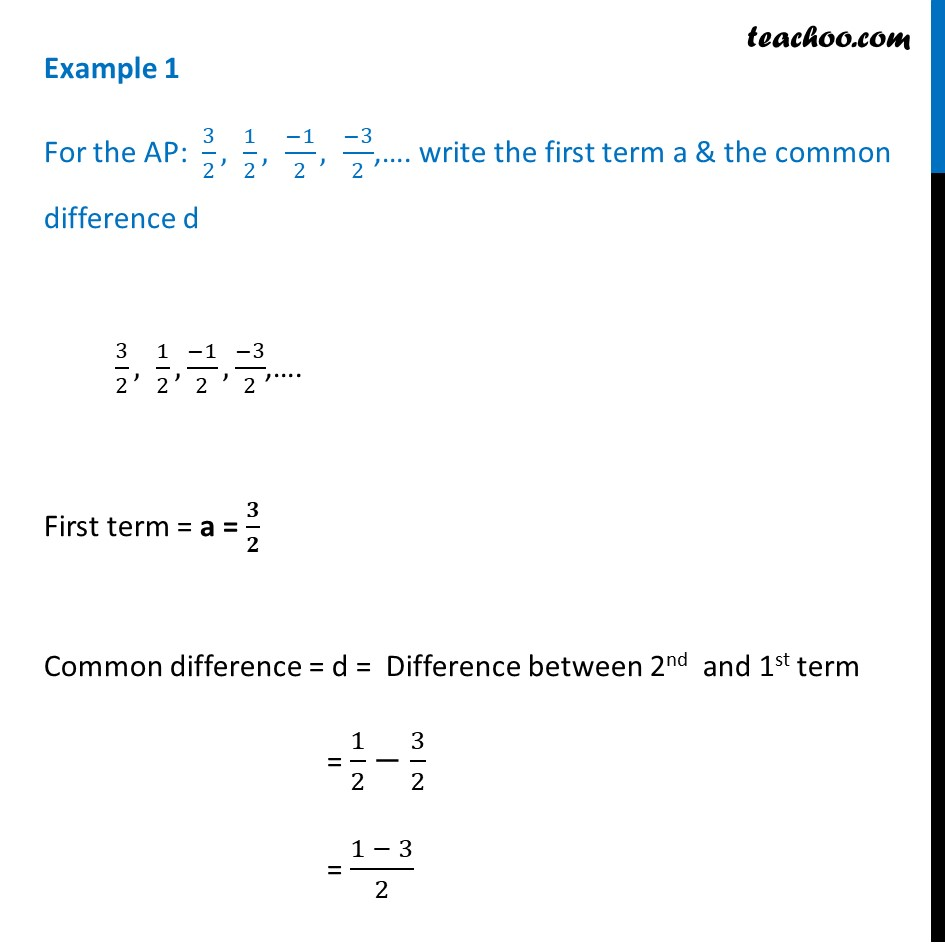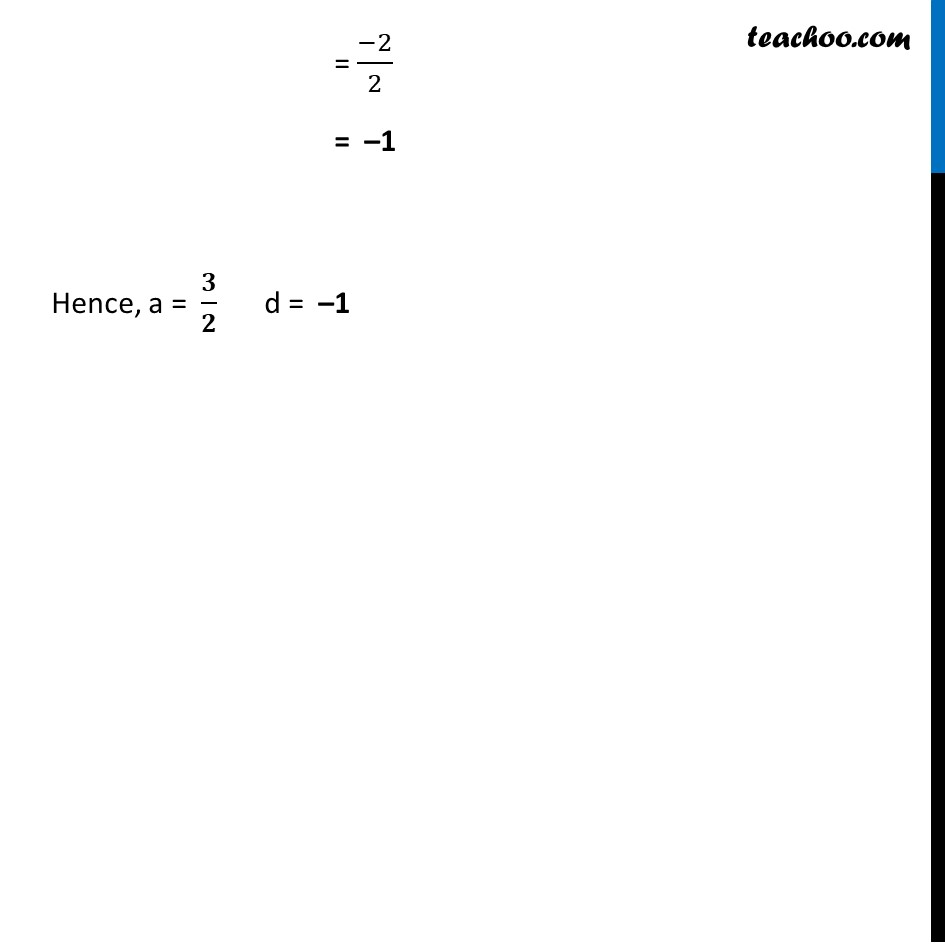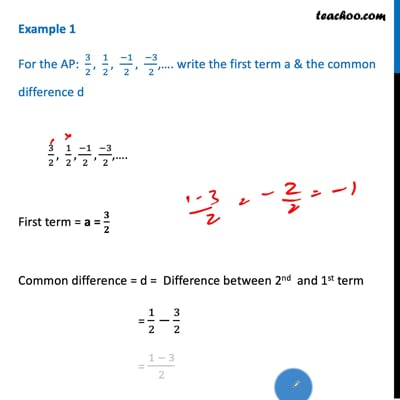Examples

Chapter 5 Class 10 Arithmetic Progressions
Serial order wiseThis video is only available for Teachoo black users

Solve all your doubts with Teachoo Black (new monthly pack available now!)

### Transcript

Example 1 For the AP: 3/2, 1/2, (−1)/2, (−3)/2,…. write the first term a & the common difference d 3/2, 1/2, (−1)/2, (−3)/2,…. First term = a = 𝟑/𝟐 Common difference = d = Difference between 2nd and 1st term = 1/2−3/2 = (1 − 3)/2 = (−2)/2 = –1 Hence, a = 𝟑/𝟐 d = –1